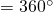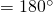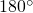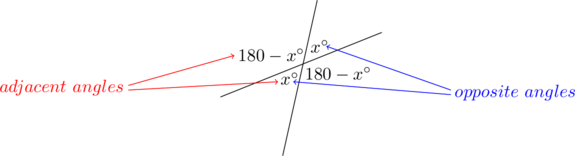## Geometry Cheat Sheet: Lines and Angles

In each Cheat Sheet, I’ll cover, as succinctly as possible, every rule you absolutely must know to solve problems in a single area found on standardized tests.

Learning these rules isn’t a substitute for developing higher-order problem-solving and strategic thinking skills; rather, it’s a necessary precondition and foundation for all of that strategizing to take place. This Cheat Sheet lists the minimum requirements to get your foot in the door. It’s the price of admission.

If you’re taking any test involving geometry, here’s what you need to know about lines and angles:

Table of Contents

• Lines and Angles
• Triangles
• Quadrilaterals
• Other Polygons
• Circles
• 3-D Geometry
• Coordinate Geometry

Total Angle Measure and Straight Angles

• One revolution• Straight angle``````

• When two lines intersect, the opposite angles are equal and the adjacent angles sum to.

``````

Parallel Lines and Opposite Interior Angles

• Given two parallel lines and a third line intersecting both, the opposite interior angles of the parallel lines are equal.
• Note: This fact, combined with the information above, means that knowing any one of the eight angles created in this situation enables determination the measures of the other seven angles.

Linesandare parallel.

``````

Tags: , , , , , ,